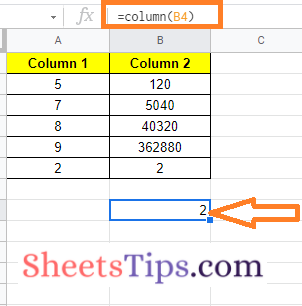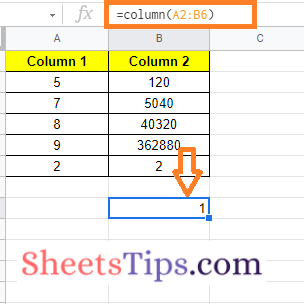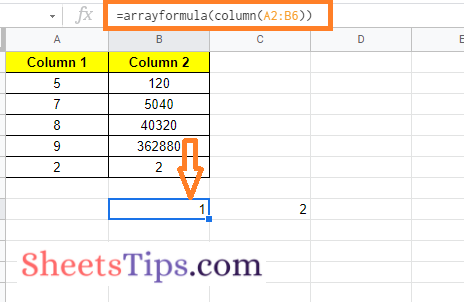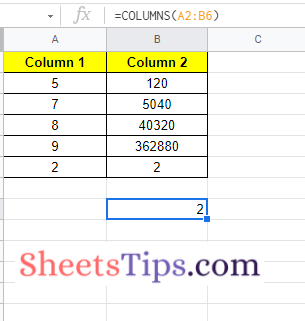# Column & Columns in Google Sheets: Using COLUMN Formula in Google Sheets

Columns and columns, though they appear to be the same thing, are two distinct functions in Google Sheets. The Column function in Google Sheets is used to return the column number of a specified cell, whereas the Columns function in the spreadsheet is used to return the total number of columns in a specified cell range or array of numbers. On this page, let us understand everything about columns and columns functions and how to use them using the Google Sheets Tips provided on this page. Read further to find out more.

## Syntax of Column and Columns Functions

The syntax of column and column functions in Google Sheets is given below:

### Column Syntax in Google Sheets

COLUMN ([cell_reference])

• Cell reference: [OPTIONAL] -By default, the formula cell] -The cell to which the column number will be returned. 1 relates to Column A.
• The location of the first column in the cell reference is returned if the cell reference is a range of more than one cell and the formula is not an array formula.

### Columns Syntax in Google Sheets

COLUMNS (range)

The cell range or column range whose count will be returned in Google Sheets.

### How to Use the Column Formula in Google Sheets?

The steps to using the column formula in a Google Spreadsheet are outlined below.

• 2nd Step: Now on the homepage, move to the cell where you want to know the column number.
• 3rd Step: Enter the formula as “Column (Cell_Range)“. In this example, I am using the formula Column (b4) since I want to know the column number of B4.
• 4th Step: Press the “Enter” key and you will see the results as shown below.### Using the COLUMN Formula for an Array of Cells

We can also use the column formula for an array of cells. This comes in handy when you want to know the column number of large cell ranges, such as Column L, AB, AC, and much more. The steps to use the column formula for the array of cells are given below:

• 1st Step: Open the Google Sheets where you want to find the column count for an array of cells.
• 2nd Step: Now move to the cell where you want to get the column number.
• 3rd Step: Enter the formula “Column (Cell range1: Cell range 2)”. In this post, I am using the formula Column (a2: b6) since I want to find the column number for the cells a2 to b6. So, according to your cell range, optimize this formula.
• 4th Step: Then press the “Enter” key and you will see the result as follows:This problem arises since the COLUMN formula takes only the first cell range number into the count. To overcome this issue, we can simply attach the array formula to the column formula. All you have to do is tweak the existing formula as “ArrayFormula (COLUMN (a2: b6)“. Once you enter this formula into Google Sheets, you will be able to see the following result.### How to Use the Columns Formula in Google Sheets?

As discussed above, a column formula is used to know the column number of the cell range. When it comes to the COLUMNS function, it is widely used to know the number of columns in a specified cell range.

The steps to using the columns formula in Google Sheets are given below: